## Ambiguous Grammar & Parse Tree-

We have discussed-

• Ambiguous Grammar generates at least one string that has more than one parse tree.
• Parse Tree is the geometrical representation of a derivation.

## Important Points-

### Point-01:

• There always exists a unique parse tree corresponding to each leftmost derivation and rightmost derivation.

 If n parse trees exist for any string w, then there will be-n corresponding leftmost derivations.n corresponding rightmost derivations.

### Point-02:

For ambiguous grammars,

• More than one leftmost derivation and more than one rightmost derivation exist for at least one string.
• Leftmost derivation and rightmost derivation represents different parse trees.

### Point-03:

For unambiguous grammars,

• A unique leftmost derivation and a unique rightmost derivation exist for all the strings.
• Leftmost derivation and rightmost derivation represents the same parse tree.

### Point-04:

• There may exist derivations for a string which are neither leftmost nor rightmost.

### Example

Consider the following grammar-

S → ABC

A → a

B → b

C → c

Consider a string w = abc.

Total 6 derivations exist for string w.

The following 4 derivations are neither leftmost nor rightmost-

#### Derivation-01:

S → ABC

→ aBC    (Using A → a)

→ aBc     (Using C → c)

→ abc     (Using B → b)

#### Derivation-02:

S → ABC

→ AbC    (Using B → b)

→ abC     (Using A → a)

→ abc     (Using C → c)

#### Derivation-03:

S → ABC

→ AbC    (Using B → b)

→ Abc     (Using C → c)

→ abc     (Using A → a)

The other 2 derivations are leftmost derivation and rightmost derivation.

### Point-05:

• Leftmost derivation and rightmost derivation of a string may be exactly same.
• In fact, there may exist a grammar in which leftmost derivation and rightmost derivation is exactly same for all the strings.

### Example

Consider the following grammar-

S → aS / ∈

The language generated by this grammar is-

L = { an , n>=0 } or a*

All the strings generated from this grammar have their leftmost derivation and rightmost derivation exactly same.

Let us consider a string w = aaa.

#### Leftmost Derivation-

S → aS

→ aaS       (Using S → aS)

→ aaaS     (Using S → aS)

→ aaa∈

→ aaa

#### Rightmost Derivation-

S → aS

→ aaS       (Using S → aS)

→ aaaS     (Using S → aS)

→ aaa∈

→ aaa

Clearly,

Leftmost derivation = Rightmost derivation

Similar is the case for all other strings.

### Point-06:

• For a given parse tree, we may have its leftmost derivation exactly same as rightmost derivation.

### Point-07:

• If for all the strings of a grammar, leftmost derivation is exactly same as rightmost derivation, then that grammar may be ambiguous or unambiguous.

### Example

Consider the following grammar-

S → aS / ∈

• This is an example of an unambiguous grammar.
• Here, each string have its leftmost derivation and rightmost derivation exactly same.

Now, consider the following grammar-

S → aS / a / ∈

• This is an example of ambiguous grammar.
• Here also, each string have its leftmost derivation and rightmost derivation exactly same.

Consider a string w = a.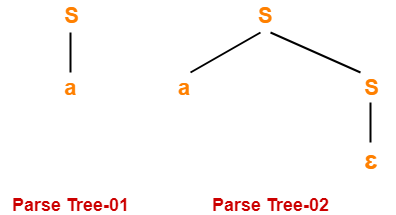Since two different parse trees exist, so grammar is ambiguous.

Leftmost derivation and rightmost derivation for parse tree-01 are-

S → a

Leftmost derivation and rightmost derivation for parse tree-02 are-

S → aS

S → a∈

S → a

Next Article- Language of Grammar

Get more notes and other study material of Theory of Automata and Computation.

Watch video lectures by visiting our YouTube channel LearnVidFun.

## Grammar Ambiguity-

Before you go through this article, make sure that you have gone through the previous article on Grammar Ambiguity.

We have discussed-

• There exists no general algorithm to remove the ambiguity from grammar.
• To check grammar ambiguity, we try finding a string that has more than one parse tree.
• If any such string exists, then the grammar is ambiguous otherwise not.

In this article, we will discuss how to convert ambiguous grammar into unambiguous grammar.

## Converting Ambiguous Grammar Into Unambiguous Grammar-

• Causes such as left recursion, common prefixes etc makes the grammar ambiguous.
• The removal of these causes may convert the grammar into unambiguous grammar.
•  However, it is not always compulsory.

### NOTE

It is not always possible to convert an ambiguous grammar into an unambiguous grammar.

## Methods To Remove Ambiguity-

The ambiguity from the grammar may be removed using the following methods-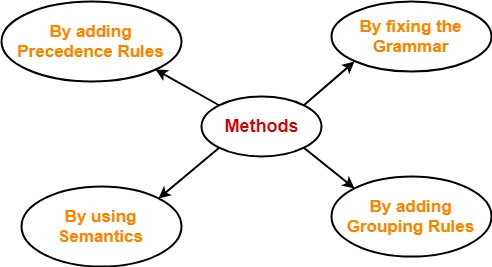• By fixing the grammar
• By using semantics and choosing the parse that makes the most sense
• By adding the precedence rules or other context sensitive parsing rules

## Removing Ambiguity By Precedence & Associativity Rules-

An ambiguous grammar may be converted into an unambiguous grammar by implementing-

• Precedence Constraints
• Associativity Constraints

These constraints are implemented using the following rules-

### Rule-01:

The precedence constraint is implemented using the following rules-

• The level at which the production is present defines the priority of the operator contained in it.
• The higher the level of the production, the lower the priority of operator.
• The lower the level of the production, the higher the priority of operator.

### Rule-02:

The associativity constraint is implemented using the following rules-

• If the operator is left associative, induce left recursion in its production.
• If the operator is right associative, induce right recursion in its production.

## Problem-01:

Convert the following ambiguous grammar into unambiguous grammar-

R → R + R / R . R / R* / a / b

where * is kleen closure and . is concatenation.

## Solution-

To convert the given grammar into its corresponding unambiguous grammar, we implement the precedence and associativity constraints.

We have-

• Given grammar consists of the following operators-

+ , . , *

• Given grammar consists of the following operands-

a , b

The priority order is-

(a , b) > * > . > +

where-

• . operator is left associative
• + operator is left associative

Using the precedence and associativity rules, we write the corresponding unambiguous grammar as-

E → E + T / T

T → T . F / F

F → F* / G

G → a / b

Unambiguous Grammar

OR

E → E + T / T

T → T . F / F

F → F* / a / b

Unambiguous Grammar

## Problem-02:

Convert the following ambiguous grammar into unambiguous grammar-

bexp → bexp or bexp / bexp and bexp / not bexp / T / F

where bexp represents Boolean expression, T represents True and F represents False.

## Solution-

To convert the given grammar into its corresponding unambiguous grammar, we implement the precedence and associativity constraints.

We have-

• Given grammar consists of the following operators-

or , and , not

• Given grammar consists of the following operands-

T , F

The priority order is-

(T , F) > not > and > or

where-

• and operator is left associative
• or operator is left associative

Using the precedence and associativity rules, we write the corresponding unambiguous grammar as-

bexp → bexp or M / M

M → M and N / N

N → not N / G

G → T / F

Unambiguous Grammar

OR

bexp → bexp or M / M

M → M and N / N

N → not N / T / F

Unambiguous Grammar

To gain better understanding about Conversion into Unambiguous Grammar-

Watch this Video Lecture

Next Article- Evaluating Expressions Based On Given Grammar

Get more notes and other study material of Theory of Automata and Computation.

Watch video lectures by visiting our YouTube channel LearnVidFun.

## Evaluating Expressions Based On Given Grammar-

There are two methods for evaluating the expressions based on given grammar-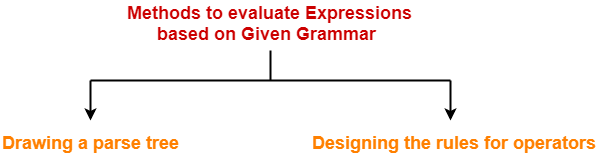1. Drawing a parse tree
2. Designing the rules for operators

### Method-01: Drawing Parse Tree-

In this method, following steps are followed-

• We draw a Parse Tree for the given expression.
• We evaluate the parse tree to get the required value of the expression.

### Drawback-

• Drawing a parse tree for large expressions is cumbersome and time taking.
• So, this method becomes difficult to follow when the expression is large.

### Method-02: Designing Rules For Operators-

In this method, following steps are followed-

• We decide the priority and associativity of operators in the grammar.
• We parenthesize the given expression.
• We evaluate the expression to get the required value.

• This method is easy to follow whether the expression is small or large.
• So, this method is preferred.

### Rules For Deciding Priority Of Operators-

For the given unambiguous grammar,

• The priority of operators is decided by checking the level at which the production is present.
• The higher the level of production, the lower the priority of operator contained in it.
• The lower the level of production, the higher the priority of operator contained in it.

### Example-

Consider the grammar-

E → E x F / F + E / F

F → F – T / T

T → id

Here,

• id has the highest priority.

(since T → id is present at the bottom most level)

• x and + operators have the least priority.

(since E → E x F / F + E are present at the top most level)

• x and + operators have the same priority.

(since E → E x F / F + E are present at the same level)

• – operator has higher priority than x and + but lesser priority than id.

(since F → F – T is present at the middle level)

So, the priority order is-

id > ( x , + )

### Rules For Deciding Associativity Of Operators-

For the given unambiguous grammar,

• The associativity of operators is decided by checking the Type Of Recursion in the production.
• If the production has left recursion, then the operator is left associative.
• If the production has right recursion, then the operator is right associative.
• If the production has both left and right recursion, then the operator is neither left associative nor right associative.

### Example-

Consider the grammar-

E → E x F / F + E / F

F → F – F / T

T → id

Here,

• x operator is left associative.

(since E → E x F has left recursion in it)

• + operator is right associative.

(since E → F + E has right recursion in it)

• F – F is neither left associative nor right associative.

(since F → F – F has both left and right recursion in it)

## Problem-01:

Consider the given grammar-

E → E + T / T

T → F x T / F

F → id

Evaluate the following expression in accordance with the given grammar-

2 + 3 x 5 x 6 + 2

## Solution-

### Method-01:

• Let us draw a parse tree for the given expression.
• Evaluating the parse tree will return the required value of the expression.

The parse tree for the given expression is-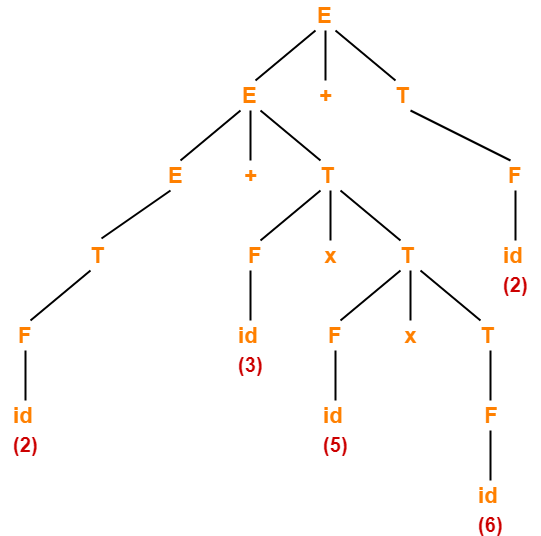On evaluating this parse tree, we get the value = 94.

### Method-02:

The priority order and associativity of operators on the basis of given grammar is-

idx > +

where-

• x operator is right associative.
• + operator is left associative.

Now, we parenthesize the given expression based on the precedence and associativity of operators as-

( 2 + ( 3 x ( 5 x 6 ) ) ) + 2

Now, we evaluate the parenthesized expression as-

= ( 2 + ( 3 x ( 5 x 6 ) ) ) + 2

= ( 2 + ( 3 x 30 ) ) + 2

= ( 2 + 90 ) + 2

= 92 + 2

= 94

## Problem-02:

Consider the given grammar-

E → E + T / E – T / T

T → T x F / T ÷ F / F

F → G ↑ F / G

G → id

Evaluate the following expression in accordance with the given grammar-

2 x 1 + 4 ↑ 2 ↑ 1 x 1 + 3

## Solution-

### Method-01:

• Let us draw a parse tree for the given expression.
• Evaluating the parse tree will return the required value of the expression.

The parse tree for the given expression is-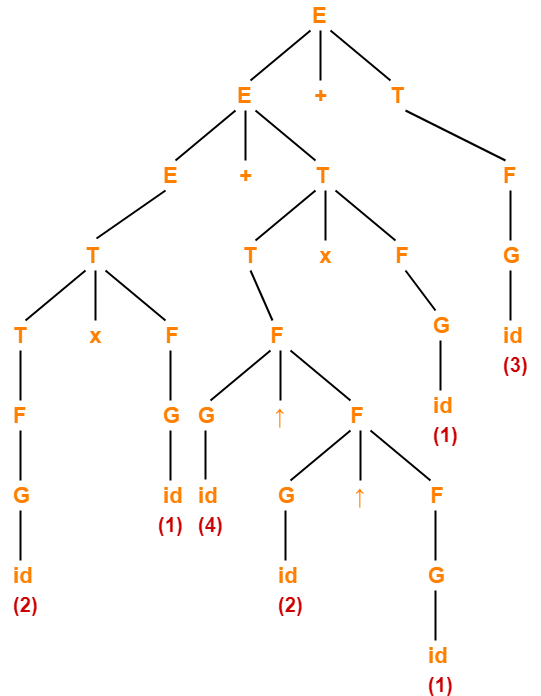On evaluating this parse tree, we get the value = 21.

### Method-02:

The priority order and associativity of operators on the basis of given grammar is-

id > ( x÷ ) > ( + , )

where-

• + , – , x , ÷ operators are left associative.
• ↑ operator is right associative

Now, we parenthesize the given expression based on the precedence and associativity of operators as-

( ( 2 x 1 ) + ( ( 4 ↑ ( 2 ↑ 1 ) ) x 1 ) ) + 3

Now, we evaluate the parenthesized expression as-

= ( ( 2 x 1 ) + ( ( 4 ↑ ( 2 ↑ 1 ) ) x 1 ) ) + 3

= ( ( 2 x 1 ) + ( ( 4 ↑ 2 ) x 1 ) ) + 3

= ( ( 2 x 1 ) + ( 16 x 1 ) ) + 3

= ( 2 + ( 16 x 1 ) ) + 3

( 2 + 16 ) + 3

= 18 + 3

= 21

## Problem-03:

Consider the given grammar-

E → E + T / E – T / T

T → T x F / T ÷ F / F

F → F ↑ G / G

G → id

Evaluate the following expression in accordance with the given grammar-

2 ↑ 1 ↑ 4 + 3 x 5 x 6 ↑ 1 + 2 ↑ 3

## Solution-

The priority order and associativity of operators on the basis of given grammar is-

id > ( x÷ ) > ( + , )

where + , – , x , ÷, ↑ operators are left associative.

Now, we parenthesize the given expression based on the precedence and associativity of operators as-

( ( ( 2 ↑ 1 ) ↑ 4 ) + ( ( 3 x 5 ) x ( 6 ↑ 1 ) ) ) + ( 2 ↑ 3 )

Now, we evaluate the parenthesized expression as-

= ( ( ( 2 ↑ 1 ) ↑ 4 ) + ( ( 3 x 5 ) x ( 6 ↑ 1 ) ) ) + ( 2 ↑ 3 )

= ( ( 2 ↑ 4 ) + ( ( 3 x 5 ) x ( 6 ↑ 1 ) ) ) + ( 2 ↑ 3 )

= ( 16 + ( ( 3 x 5 ) x ( 6 ↑ 1 ) ) ) + ( 2 ↑ 3 )

= ( 16 + ( ( 3 x 5 ) x 6 ) ) + ( 2 ↑ 3 )

= ( 16 + ( ( 3 x 5 ) x 6 ) ) + 8

= ( 16 + ( 15 x 6 ) ) + 8

( 16 + 90 ) + 8

= 106 + 8

= 114

## Problem-04:

Consider the given grammar-

E → E ↑ T / T

T → T + F / F

F → G – F / G

G → id

Evaluate the following expression in accordance with the given grammar-

2 ↑ 1 ↑ 3 + 5 – 6 – 8 – 5 + 10 + 11 ↑ 2

## Solution-

The priority order and associativity of operators on the basis of given grammar is-

id >  > + >

where-

• + , ↑ operators are left associative.
• – is right associative.

Now, we parenthesize the given expression based on the precedence and associativity of operators as-

( ( 2 ↑ 1 ) ↑ ( ( ( 3 + ( 5 – ( 6 – ( 8 – 5 ) ) ) ) + 10 ) + 11 ) ) ↑ 2

Now, we evaluate the parenthesized expression as-

= ( ( 2 ↑ 1 ) ↑ ( ( ( 3 + ( 5 – ( 6 – ( 8 – 5 ) ) ) ) + 10 ) + 11 ) ) ↑ 2

= ( ( 2 ↑ 1 ) ↑ ( ( ( 3 + ( 5 – ( 6 – 3 ) ) ) + 10 ) + 11 ) ) ↑ 2

= ( ( 2 ↑ 1 ) ↑ ( ( ( 3 + ( 5 – 3 ) ) + 10 ) + 11 ) ) ↑ 2

= ( ( 2 ↑ 1 ) ↑ ( ( ( 3 + 2 ) + 10 ) + 11 ) ) ↑ 2

= ( ( 2 ↑ 1 ) ↑ ( ( 5 + 10 ) + 11 ) ) ↑ 2

= ( ( 2 ↑ 1 ) ↑ ( 15 + 11 ) ) ↑ 2

= ( ( 2 ↑ 1 ) ↑ 26 ) ↑ 2

= ( 2 ↑ 26 ) ↑ 2

= (226) ↑ 2

= (226)2

## Problem-05:

Consider the given priority order of operators-

id > ( x÷ ) > ( + , )

Given all the operators are left associative, construct the corresponding grammar.

## Solution-

We apply the rules learnt above in reverse direction to obtain the required grammar.

The corresponding grammar is-

E → E + T / E – T / T

T → T x F / T ÷ F / F

F → id

## Problem-06:

Consider the given priority order of operators-

id > ( x÷ ) > ( + , )

Given all the operators are left associative, construct the corresponding grammar.

## Solution-

The corresponding grammar is-

E → E + T / E – T / T

T → T x F / T ÷ F / F

F → G ↑ F / G

G → id

Next Article- Important Points For Exams

Get more notes and other study material of Theory of Automata and Computation.

Watch video lectures by visiting our YouTube channel LearnVidFun.

## Grammar in Automata-

Before you go through this article, make sure that you have gone through the previous article on Types of Grammar in Automata.

On the basis of number of strings, grammars are classified as-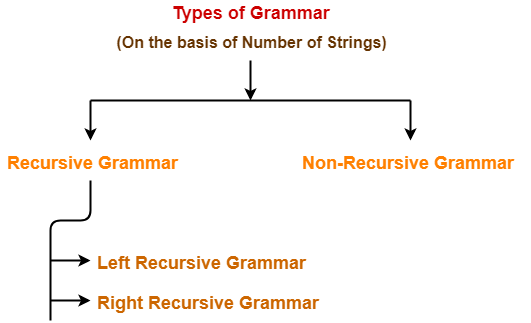1. Recursive Grammar
2. Non-Recursive Grammar

## 1. Recursive Grammar-

• A grammar is said to be recursive if it contains at least one production that has the same variable at both its LHS and RHS.

OR

• A grammar is said to be recursive if and only if it generates infinite number of strings.

A recursive grammar may be either-

1. Left recursive grammar
2. Right recursive grammar

### A) Left Recursive Grammar-

• A recursive grammar is said to be left recursive if the leftmost variable of RHS is same as variable of LHS.

OR

• A recursive grammar is said to be left recursive if it has Left Recursion.

### Example-

S → Sa / b

(Left Recursive Grammar)

### B) Right Recursive Grammar-

• A recursive grammar is said to be right recursive if the rightmost variable of RHS is same as variable of LHS.

OR

• A recursive grammar is said to be right recursive if it has right recursion.

### Example-

S → aS / b

(Right Recursive Grammar)

## 2. Non-Recursive Grammar-

• A grammar is said to be non-recursive if it contains no production that has the same variable at both its LHS and RHS.

OR

• A grammar is said to be non-recursive if and only if it generates finite number of strings.

### NOTE

A non-recursive grammar has neither left recursion nor right recursion.

### Example-

S → aA / bB

A → a / b

B → c / d

(Non-Recursive Grammar)

The language generated from this grammar is L = { aa , ab , bc , bd }

Since the grammar generates finite number of strings, therefore it is a non-recursive grammar.

## Important Notes-

### Note-01:

The grammar which is either left recursive or right recursive is always unambiguous.

Examples-

• S → aS / b   (Unambiguous Grammar)
• S → Sa / b   (Unambiguous Grammar)

### Note-02:

The grammar which is both left recursive and right recursive is always ambiguous.

Example-

E → E + E / E – E / E x E / id

(Ambiguous Grammar)

### Note-03:

• Left recursive grammar is not suitable for Top down parsers.
• This is because it makes the parser enter into an infinite loop.
• To avoid this situation, it is converted into its equivalent right recursive grammar.
• This is done by eliminating left recursion from the left recursive grammar.

### Note-04:

• The conversion of left recursive grammar into right recursive grammar and vice-versa is decidable.

To gain better understanding about Recursive Grammar,

Watch this Video Lecture

Next Article- Ambiguous Vs Unambiguous Grammar

Get more notes and other study material of Theory of Automata and Computation.

Watch video lectures by visiting our YouTube channel LearnVidFun.

## Grammar Ambiguity-

Before you go through this article, make sure that you have gone through the previous article on Ambiguous Grammar.

• There exists no algorithm to check whether any given grammar is ambiguous or not.
• This general decision problem is undecidable-

“Whether a grammar is ambiguous or not?”

• This is because it can be shown that this problem is equivalent to Post Correspondence Problem.

## General Approach To Check Grammar Ambiguity-

To check whether a given grammar is ambiguous or not, we follow the following steps-

### Step-01:

We try finding a string from the Language of Grammar such that for the string there exists more than one-

• parse tree
• or derivation tree
• or syntax tree
• or leftmost derivation
• or rightmost derivation

### Step-02:

If there exists at least one such string, then the grammar is ambiguous otherwise unambiguous.

## Problem-01:

Check whether the given grammar is ambiguous or not-

S → SS

S → a

S → b

## Solution-

Let us consider a string w generated by the given grammar-

w = abba

Now, let us draw parse trees for this string w.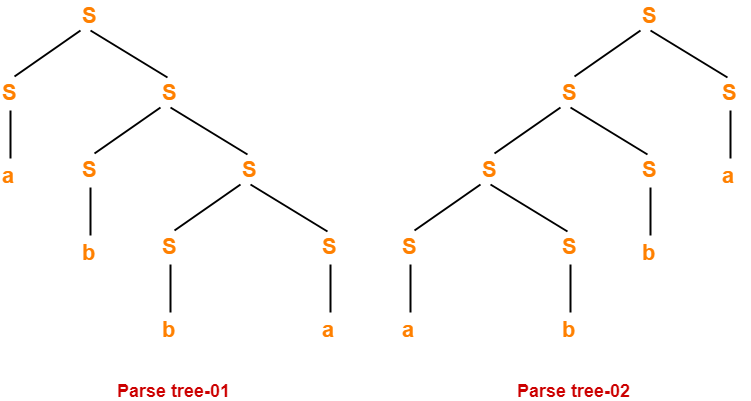Since two different parse trees exist for string w, therefore the given grammar is ambiguous.

## Problem-02:

Check whether the given grammar is ambiguous or not-

S → A / B

A → aAb / ab

B → abB / ∈

## Solution-

Let us consider a string w generated by the given grammar-

w = ab

Now, let us draw parse trees for this string w.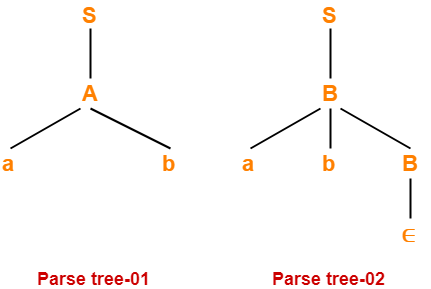Since two different parse trees exist for string w, therefore the given grammar is ambiguous.

## Problem-03:

Check whether the given grammar is ambiguous or not-

S → AB / C

A → aAb / ab

B → cBd / cd

D → bDc / bc

## Solution-

Let us consider a string w generated by the given grammar-

w = aabbccdd

Now, let us draw parse trees for this string w.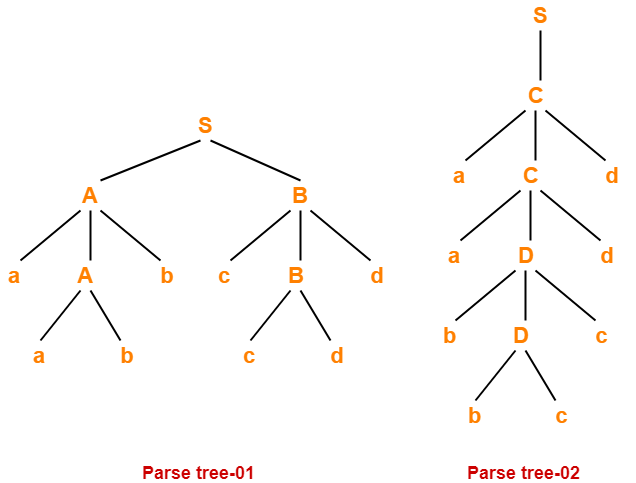Since two different parse trees exist for string w, therefore the given grammar is ambiguous.

## Problem-04:

Check whether the given grammar is ambiguous or not-

S → AB / aaB

A → a / Aa

B → b

## Solution-

Let us consider a string w generated by the given grammar-

w = aab

Now, let us draw parse trees for this string w.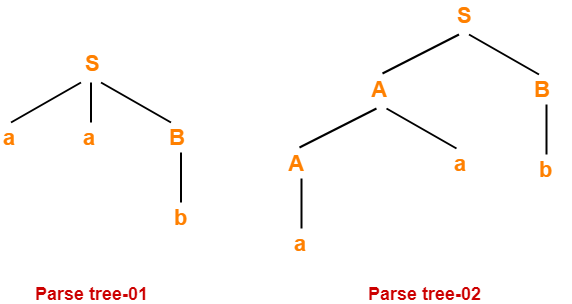Since two different parse trees exist for string w, therefore the given grammar is ambiguous.

## Problem-05:

Check whether the given grammar is ambiguous or not-

S → a / abSb / aAb

A → bS / aAAb

## Solution-

Let us consider a string w generated by the given grammar-

w = abababb

Now, let us draw parse trees for this string w.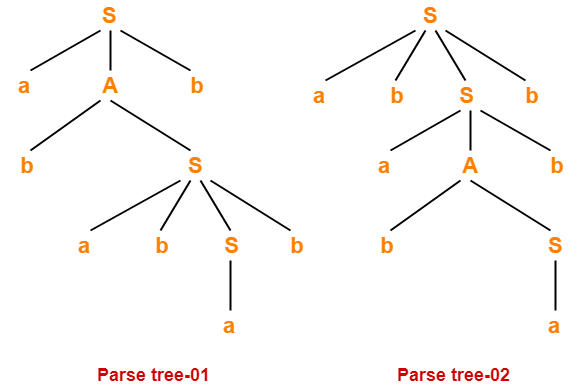Since two different parse trees exist for string w, therefore the given grammar is ambiguous.

## Problem-06:

Check whether the given grammar is ambiguous or not-

E → E + T / T

T → T x F / F

F → id

## Solution-

• There exists no string belonging to the language of grammar which has more than one parse tree.
• Since a unique parse tree exists for all the strings, therefore the given grammar is unambiguous.

## Problem-07:

Check whether the given grammar is ambiguous or not-

S → aSbS / bSaS / ∈

## Solution-

Let us consider a string w generated by the given grammar-

w = abab

Now, let us draw parse trees for this string w.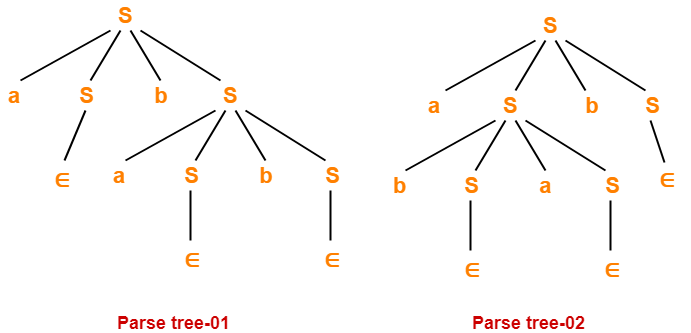Since two different parse trees exist for string w, therefore the given grammar is ambiguous.

## Problem-08:

Check whether the given grammar is ambiguous or not-

R → R + R / R . R / R* / a / b

## Solution-

Let us consider a string w generated by the given grammar-

w = ab + a

Now, let us draw parse trees for this string w.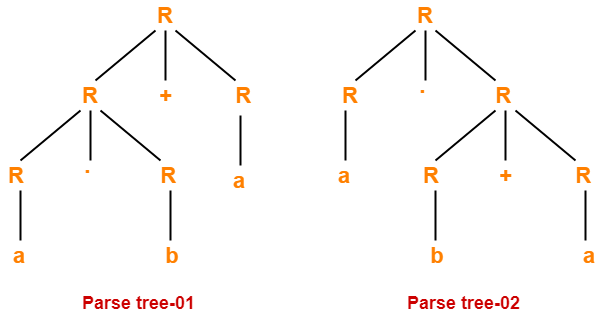Since two different parse trees exist for string w, therefore the given grammar is ambiguous.

To gain better understanding about Grammar Ambiguity,

Watch this Video Lecture

Next Article- Converting Ambiguous into Unambiguous Grammar

Get more notes and other study material of Theory of Automata and Computation.

Watch video lectures by visiting our YouTube channel LearnVidFun.